# Construction of Tangents to a Circle - Constructions, CBSE, Class 10, Mathematics Notes | Study Additional Documents & Tests for Class 10 - Class 10

## Class 10: Construction of Tangents to a Circle - Constructions, CBSE, Class 10, Mathematics Notes | Study Additional Documents & Tests for Class 10 - Class 10

The document Construction of Tangents to a Circle - Constructions, CBSE, Class 10, Mathematics Notes | Study Additional Documents & Tests for Class 10 - Class 10 is a part of the Class 10 Course Additional Documents & Tests for Class 10.
All you need of Class 10 at this link: Class 10

CONSTRUCTION OF TANGENTS TO A CIRC LE

(a) If a point lies inside a circle, we can not draw any tangent to the circle i.e., No tangent is possible in this case.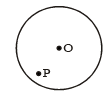If a point lies on the circle, then there is only one tangent to the circle at this point. The tangent to a circle at any point is perpendicular to the radius passing through the point of contact.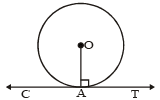Two tangents are drawn from an external point to circle, they are equal in length.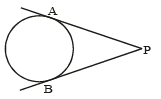Construction 4 : Draw a circle of radius 5 cm. From a point 8 cm away from its centre, construct pair of tangents to the circle measure their lengths.
Sol.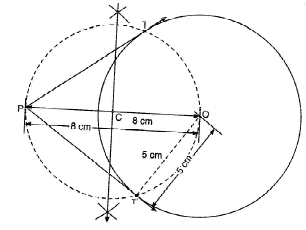Steps of Construction :
Step-1 : Draw a circle with radius 5 cm whose centre is O.
Step-2 : Take a point P at a distance 8 cm from the centre O such that OP = 8cm.
Step-3 : Bisect the line segment OP at the point C such that OC =CP =4 cm.
Step-4 : Taking C as centre and OC as arc, draw a dotted circle to intersect the given circle at the points T and T'.
Step-5 : Join PT and PT'
PT and PT' are the required pair of tangents to the circle.
By measurement we obtain PT = PT' = 6.2 cm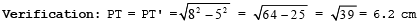Construction 5. Construct a tangent to a circle of radius 4 cm from a point on the concentric circle of radius 6 cm and measure its length. Also verify the measurement by actual calculation.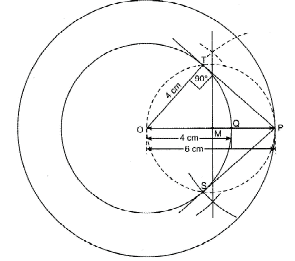Steps of Construction:
Step 1 : Draw two concentric circles with centre O and radii 4 cm and 6 cm such that OP = 6 cm, OQ = 4 cm.
Step 2 : Join OP and bisect it at M. i.e. M is the mid-point of OP i.e. OM = PM = 3 cm.
Step 3 : Taking M as centre with OM as radius draw a circle intersecting the smaller circle in two points namely T and S.
Step 4 : Join PT and PS.
PT and PS are the required tangents from a point P to the smaller circle, whose radius is 4 cm. By measurement: PT = 4.5 cm.
Verification. OTP is right D at T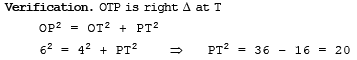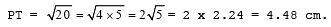The document Construction of Tangents to a Circle - Constructions, CBSE, Class 10, Mathematics Notes | Study Additional Documents & Tests for Class 10 - Class 10 is a part of the Class 10 Course Additional Documents & Tests for Class 10.
All you need of Class 10 at this link: Class 10Use Code STAYHOME200 and get INR 200 additional OFF

## Additional Documents & Tests for Class 10

5 videos|196 docs|42 tests

Track your progress, build streaks, highlight & save important lessons and more!

,

,

,

,

,

,

,

,

,

,

,

,

,

,

,

,

,

,

,

,

,

,

,

,

,

,

,

,

,

,

;